Home | | Transforms and Partial Differential Equations | Solution of the wave equation

# Solution of the wave equation

Let y = X(x) . T(t) be the solution of (1), where ‚ÄûX‚Äü is a function of ‚Äûx‚Äü only and ‚ÄûT‚Äü is a function of ‚Äût‚Äü only.

Solution of the wave equation

The wave equation isLet y = X(x) . T(t) be the solution of (1), where ‚ÄûX‚Äü is a function of ‚Äûx‚Äü only and ‚ÄûT‚Äü is a function of ‚Äût‚Äü only.Of these three solutions, we have to select that particular solution which suits the physical nature of the problem and the given boundary conditions. Since we are dealing with problems on vibrations of strings, ‚Äûy‚Äü must be a periodic function of ‚Äûx‚Äü and ‚Äût‚Äü.Now the left side of (2) is a function of ‚Äûx‚Äü only and the right side is a function of ‚Äût‚Äü only.

Since ‚Äûx‚Äü and ‚Äût‚Äü are independent variables, (2) can hold good only if each side is equal to a constant.

Hence the solution must involve trigonometric terms.

Therefore, the solution given by (5),

i.e,     y = (c5 coslx  + c6 sin lx) (c7 cosalt+ c8 sin alt)

is the only suitable solution of the wave equation.

llustrative Examples.

Example 1

If a string of length ‚Ñì is initially at rest in equilibrium position and each of its points is given the velocitySolution

The displacement y(x,t) is given by the equationThe boundary conditions are

i.           y(0,t) = 0, for t ¬≥0.

ii.    y(‚Ñì,t)  ¬≥0.=   0,   for     t

y(x,0) = 0, for 0 ¬£¬£‚Ñì.Since the vibration of a string is periodic, therefore, the solution of (1) is of the form

y(x,t) = (Acoslx + Bsinlx)(Ccoslat + Dsinlat) ------------(2)

Using (i) in (2) , we get

0 = A(Ccoslat + Dsinlat) , for all t ¬≥0.

Therefore,            A = 0

Hence equation (2) becomes

y(x,t) = B sinlx(Ccoslat + Dsinlat) ------------ (3)

Using (ii) in (3), we get

0 = Bsinl‚Ñì   (Ccoslat+Dsinlat), for all  t ¬≥0, which gives l‚Ñì = np

Hence,         l= np / l , n being an integer.Using condition (iv) in the above equation, we getExample 2

A tightly stretched string with fixed end points x = 0 & x = ‚Ñì is initially at rest in its equilibrium position . If it is set vibrating by giving to each of its points a  velocity

¬∂y/¬∂t    = kx(‚Ñì-x) at t = 0. Find the displacement y(x,t).

Solution

The displacement y(x,t) is given by the equationThe boundary conditions areExample 3

A tightly stretched string with fixed end points x = 0 & x = ‚Ñì is initially in a position given by y(x,0) = y0sin3(px/‚Ñì). If it is released from this position, find the displacement y at any time and at any distance from the end x = 0 .

Solution

The displacement y(x,t) is given by the equationThe boundary conditions are

(i)     y(0,t) = 0,  "¬≥0.

(ii)                                     y("t‚Ñì¬≥,t)0.     =   0,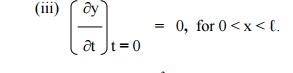(iv)  y(x,0) = y0 sin3((px/‚Ñì),   for   0   <   x   <   ‚Ñì.

The suitable solution of (1) is given by

y(x,t) = (Acoslx + Bsinlx)(Ccoslat + Dsinlat) ------------(2)

Using (i) and (ii) in (2) , we get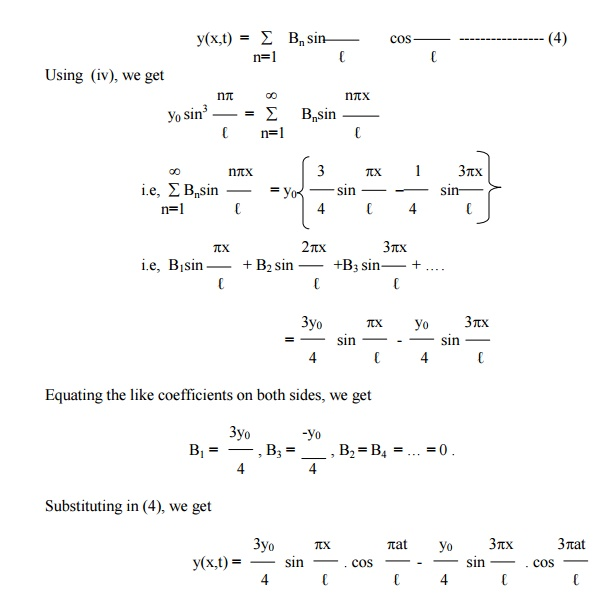Example 4

A string is stretched & fastened to two points x = 0 and x = ‚Ñì apart. Motion is started by displacing the string into the form y(x,0) = k(‚Ñìx-x2) from which it is released at time t = 0. Find the displacement y(x,t).

Solution

The displacement y(x,t) is given by the equationThe boundary conditions are

(i)     y(0,t) = 0,  "¬≥0.

(ii)   y(‚Ñì,t) "¬≥=0.  0,The suitable solution of (1) is given by

y(x,t) = (Acoslx + Bsinlx)(Ccoslat + Dsinlat) ------------(2)

Using    (i) and (ii) in (2) , we get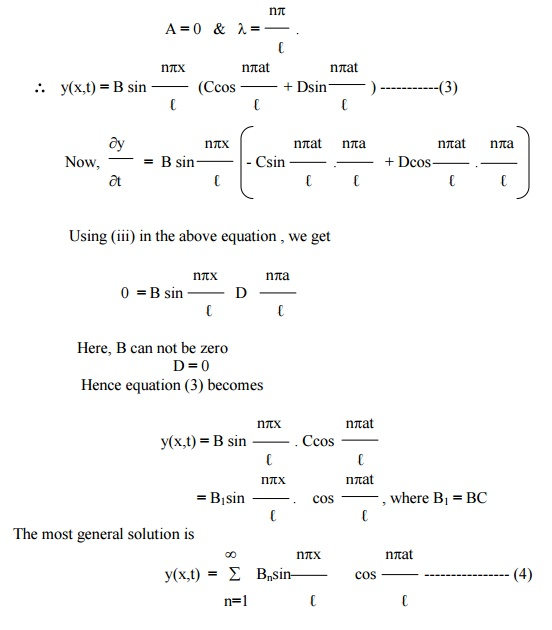Example 5

A uniform elastic string of length 2‚Ñì is fastened at both ends. The midpoint of the string is taken to the height ‚Äûb‚Äü and then released from rest in  that position . Find the displacement of the string.

Solution

The displacement y(x,t) is given by the equation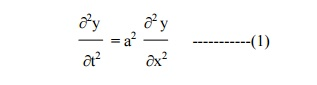The suitable solution of (1) is given by

y(x,t) = (Acoslx + Bsinlx)(Ccoslat + Dsinlat)      ------------(2)

The boundary conditions are

(i)     y(0,t) = 0,  "¬≥0.

(ii)   y(‚Ñì,t) "¬≥=0.  0,[Since,   equation   of   OA   is(y- b)/(oy-b)== (x(b/-‚Ñì)/(2‚Ñì-‚Ñì)x)]‚Ñì

Using conditions (i) and (ii) in (2), we getHere B can not be zero, therefore      D = 0.

Hence equation (3) becomesExample 6

A tightly stretched string with fixed end points x = 0 & x = ‚Ñì is initially in  the position y(x,0) = f(x). It is set vibrating by giving to each of its points a  velocity   ¬∂y/¬∂t = g(x) at t = 0 . Find the displacement y(x,t) in the form of Fourier series.

Solution

The displacement y(x,t) is given by the equation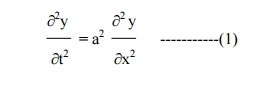The boundary conditions are

(i)     y(0,t) = 0,  "¬≥0.

(ii)   y(‚Ñì,t) "¬≥=0.  0,

(iii) y(x,0) = f(x) , for 0 ¬£¬£‚Ñì.=g(x) , for 0 ¬£¬£‚Ñì. ¬∂t t

The solution of equation .(1) is given by

y(x,t) = (Acoslx + Bsinlx)(Ccoslat + Dsinlat) ------------(2)

where A, B, C, D are constants.

Applying conditions (i) and (ii) in (2), we have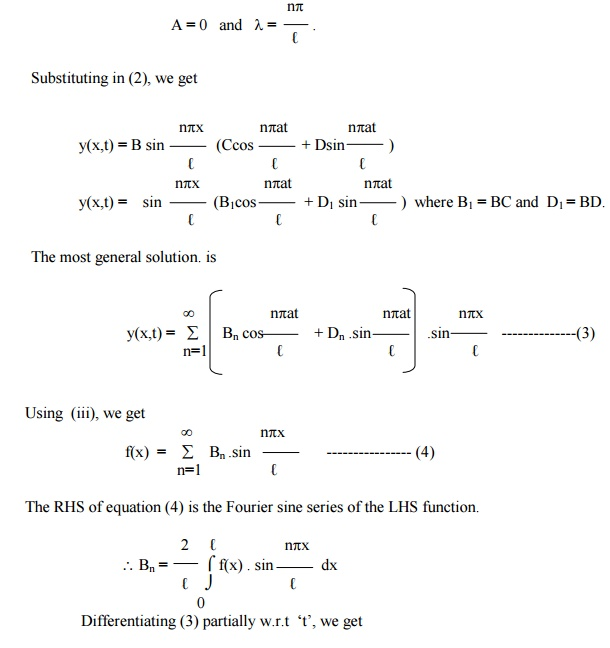Substituting the values of Bn and Dn in (3), we get the required solution of the given equation.

Exercises

(1) Find the solution of the equation of a vibrating string of   length   '‚Ñì',   satisfying the conditions

y(0,t) = y(‚Ñì,t) = 0 and y = f(x), ¬∂y/ ¬∂t = 0 at t = 0.

(2) A taut string of length 20 cms. fastened at both ends is displaced from its position of equilibrium, by imparting to each of its points an initial velocity given by

v = x      in 0 ¬£¬£10

= 20 -x  in 10 ¬£¬£20,

‚Äûx‚Äü being the distance from one end. Determine the displacement at any subsequent time.

(3) Find the solution of the wave equation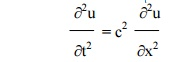corresponding to the triangular initial deflection f(x ) = (2k/ ‚Ñì)   x   where 0<x<‚Ñì/2

= (2k/ ‚Ñì) ‚Äìx)(‚Ñì-x)       where ‚Ñì/2<x<‚Ñì,

and initial velocity zero.

(4) A tightly stretched string with fixed end points x = 0 and x = ‚Ñì is initially at rest in its equilibrium position. If it is set vibrating by giving to each of its points a velocity ¬∂y/ ¬∂= f(x)

at t = 0. Find the displacement y(x,t).

(5) Solve the following boundary value problem of vibration of string

i.           y(0,t) = 0

ii.           y(‚Ñì,t)   =   0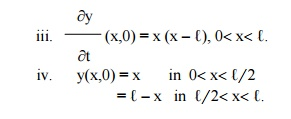(6) A tightly stretched string with fixed end points x = 0 and x = ‚Ñì is initially in a position given by y(x,0) = k( sin(px/ ‚Ñì) ‚Äì sin( 2px/ ‚Ñì)). If it is released from rest, find the displacement of ‚Äûy‚Äü at any distance ‚Äûx‚Äü from one end at any time "t‚Äü.

Study Material, Lecturing Notes, Assignment, Reference, Wiki description explanation, brief detail
Mathematics (maths) : Applications of Partial Differential Equations : Solution of the wave equation |